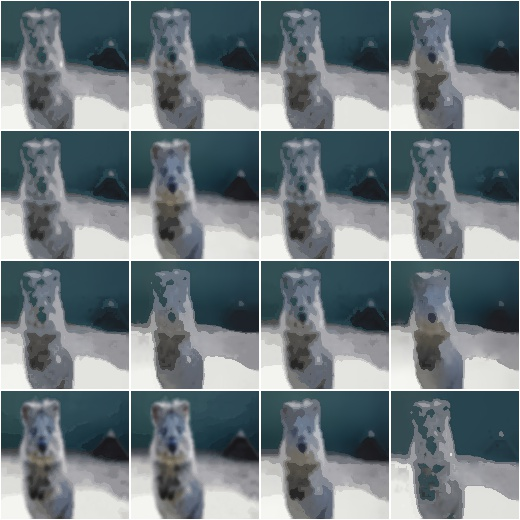# augmenters.blur¶

## GaussianBlur¶

Augmenter to blur images using gaussian kernels.

API link: `GaussianBlur`

Example. Blur each image with a gaussian kernel with a sigma of `3.0`:

```import imgaug.augmenters as iaa
aug = iaa.GaussianBlur(sigma=(0.0, 3.0))
```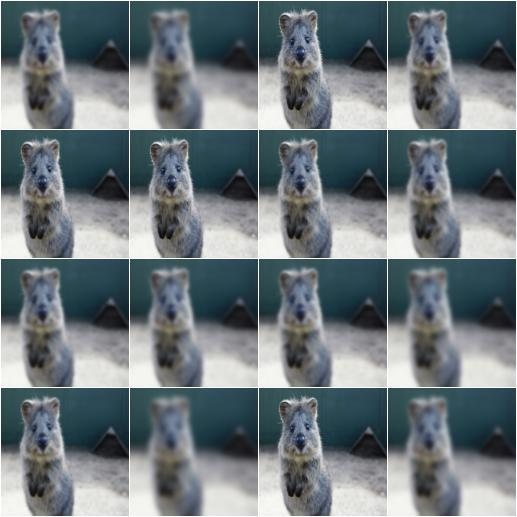## AverageBlur¶

Blur an image by computing simple means over neighbourhoods.

API link: `AverageBlur`

Example. Blur each image using a mean over neihbourhoods that have a random size between 2x2 and 11x11:

```import imgaug.augmenters as iaa
aug = iaa.AverageBlur(k=(2, 11))
```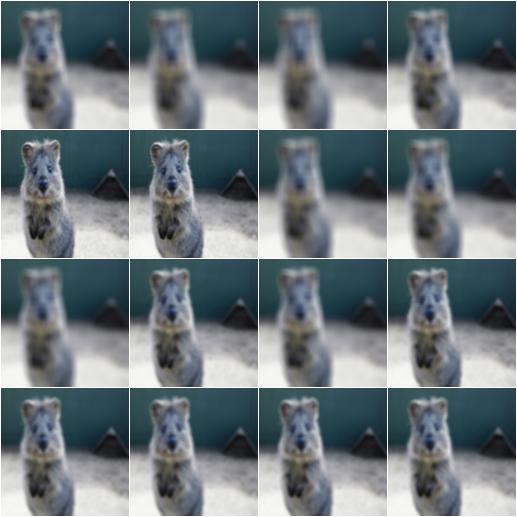Example. Blur each image using a mean over neihbourhoods that have random sizes, which can vary between 5 and 11 in height and 1 and 3 in width:

```aug = iaa.AverageBlur(k=((5, 11), (1, 3)))
```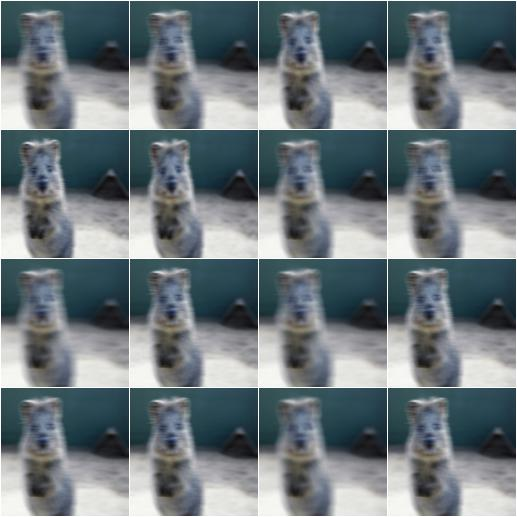## MedianBlur¶

Blur an image by computing median values over neighbourhoods.

API link: `MedianBlur`

Example. Blur each image using a median over neihbourhoods that have a random size between 3x3 and 11x11:

```import imgaug.augmenters as iaa
aug = iaa.MedianBlur(k=(3, 11))
```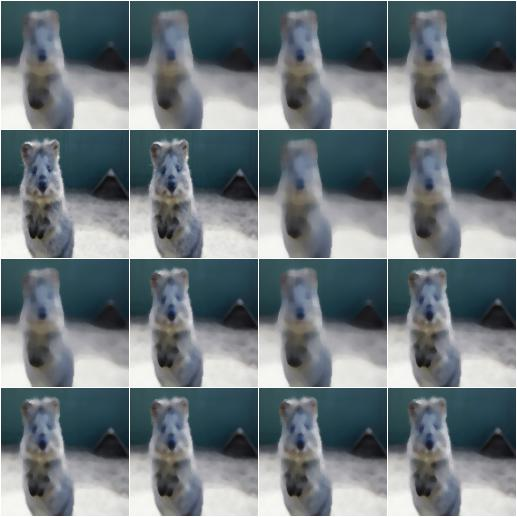## BilateralBlur¶

Blur/Denoise an image using a bilateral filter.

Bilateral filters blur homogenous and textured areas, while trying to preserve edges.

API link: `BilateralBlur`

Example. Blur all images using a bilateral filter with a max distance sampled uniformly from the interval `[3, 10]` and wide ranges for sigma_color and sigma_space:

```import imgaug.augmenters as iaa
aug = iaa.BilateralBlur(
d=(3, 10), sigma_color=(10, 250), sigma_space=(10, 250))
```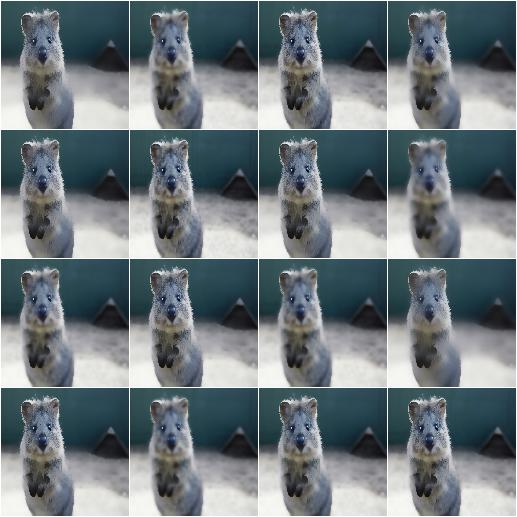## MotionBlur¶

Blur images in a way that fakes camera or object movements.

API link: `MotionBlur`

Example. Apply motion blur with a kernel size of `15x15` pixels to images:

```import imgaug.augmenters as iaa
aug = iaa.MotionBlur(k=15)
```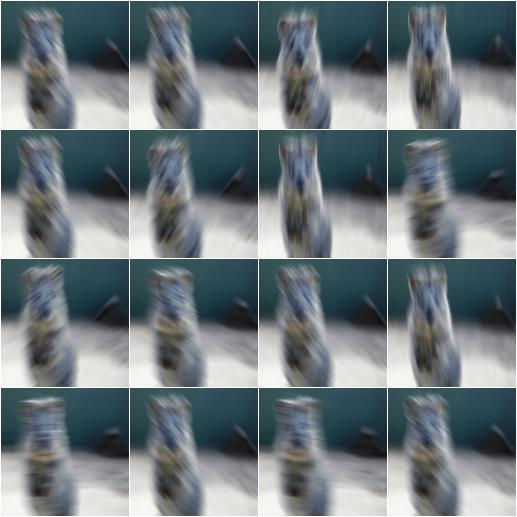Example. Apply motion blur with a kernel size of `15x15` pixels and a blur angle of either `-45` or `45` degrees (randomly picked per image):

```aug = iaa.MotionBlur(k=15, angle=[-45, 45])
```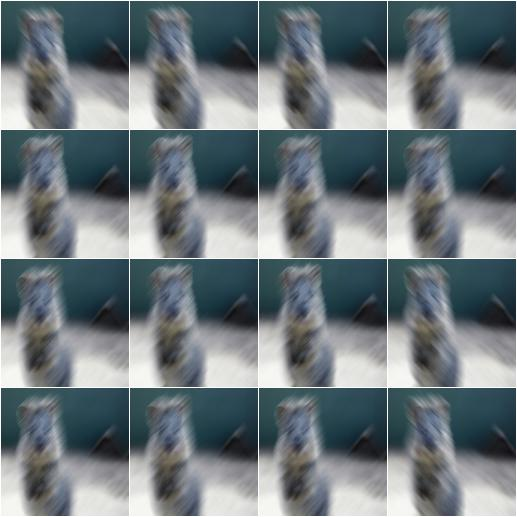## MeanShiftBlur¶

Apply a pyramidic mean shift filter to each image.

See also `blur_mean_shift_()` for details.

This augmenter expects input images of shape `(H,W)` or `(H,W,1)` or `(H,W,3)`.

Note

This augmenter is quite slow.

API link: `MeanShiftBlur`

Example. Create a mean shift blur augmenter:

```import imgaug.augmenters as iaa
aug = iaa.MeanShiftBlur()
```